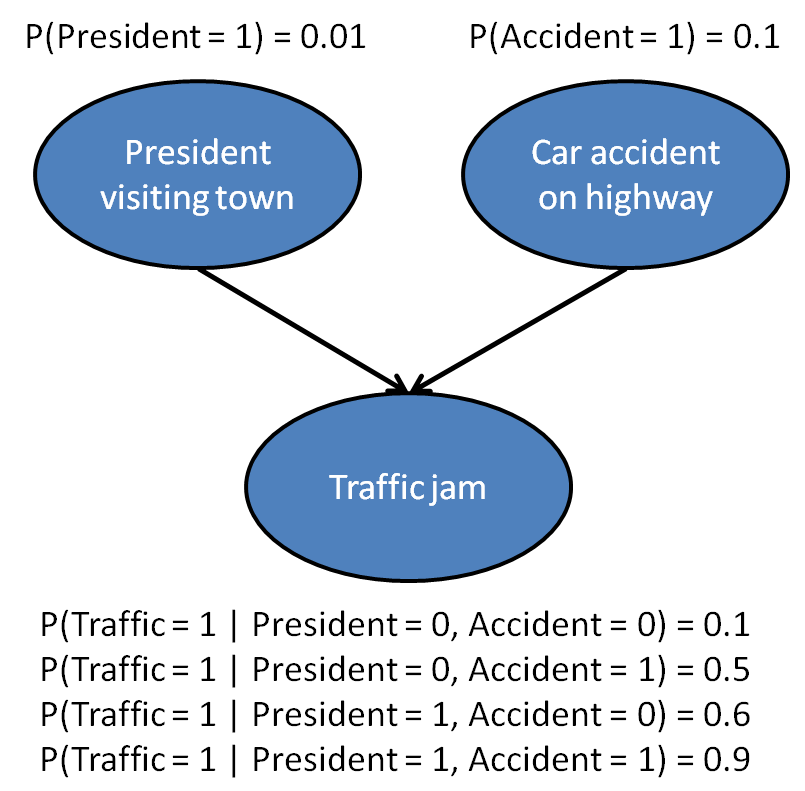Since it took me a considerable amount of time to come up with how to answer this question to Stanford University’s Probabilistic Graphical Models 1: Representation course, I decided I would be posting it online. Not because I want people to copy and paste it to get a full grade, but because, for a couple of hours, I wanted the course to give me a more thorough explanation on how to approach the problem.

Consider the following model for traffic jams in a small town, which we assume can be caused by a car accident, or by a visit from the president (and the accompanying security motorcade).Created by Stanford University.

Calculate P(Accident = 1 | Traffic = 1) and P(Accident = 1 | Traffic = 1, President = 1). Separate your answers with a space, e.g., an answer of

0.15 0.25

means that P(Accident = 1 | Traffic = 1) = 0.15 and P(Accident = 1 | Traffic = 1, President = 1) = 0.25. Round your answers to two decimal places and write a leading zero, like in the example above.

## What is given

• President visiting town

$P(P=1) = 0.01 \\ P(P=0) = 1 - P(P=1) = 0.99$

• Car accident on highway

$P(A=1) = 0.1 \\ P(A=0) = 1 - P(A=1) = 0.9$

• Traffic jam

$P(T=1 \mid P=0, A=0) = 0.1 \\ P(T=1 \mid P=0, A=1) = 0.5 \\ P(T=1 \mid P=1, A=0) = 0.6 \\ P(T=1 \mid P=1, A=1) = 0.9$

• Given it was observed a traffic jam, what are the odds of having a car accident on highway

\begin{aligned} P(A=1 \mid T=1) &= \frac{P(A=1 , T=1)}{P(T=1)} \\ &= \frac{0.0504}{0.1449} \\ &= 0.3478261 \\ &\approx 0.35 \end{aligned}

• Given it was observed a traffic jam and the president visit to town, what are the odds of having a car accident on highway

\begin{aligned} P(A=1 \mid T=1, P=1) &= \frac{P(A=1 , T=1, P=1)}{P(T=1, P=1)} \\ &= \frac{0.0009}{0.0063} \\ &= 0.1428571 \\ &\approx 0.14 \end{aligned}

### Development

\begin{aligned} P(A=1 , T=1) &= P(P=0, A=1 , T=1) + P(P=1, A=1 , T=1) \\ &= 0.0495 + 0.0009 \\ &= 0.0504 \end{aligned}

\begin{aligned} P(P=0, A=1 , T=1) &= P(P=0) \times P(A=1) \times P(T=1 \mid P=0, A=1) \\ &= 0.99 \times 0.1 \times 0.5 \\ &= 0.0495 \end{aligned}

\begin{aligned} P(P=1, A=1 , T=1) &= P(P=1) \times P(A=1) \times P(T=1 \mid P=1, A=1) \\ &= 0.01 \times 0.1 \times 0.9 \\ &= 0.0009 \end{aligned}

\begin{aligned} P(T=1) &= P(T=1, P=0, A=0) + P(T=1, P=0, A=1) + P(T=1, P=1, A=0) + P(T=1, P=1, A=1) \\ &= 0.0891 + 0.0495 + 0.0054 + 0.0009 \\ &= 0.1449 \end{aligned}

\begin{aligned} P(T=1, P=0, A=0) &= P(P=0) \times P(A=0) \times P(T=1 \mid P=0, A=0) \\ &= 0.99 \times 0.9 \times 0.1 \\ &= 0.0891 \end{aligned}

\begin{aligned} P(T=1, P=0, A=1) &= P(P=0) \times P(A=1) \times P(T=1 \mid P=0, A=1) \\ &= 0.99 \times 0.1 \times 0.5 \\ &= 0.0495 \end{aligned}

\begin{aligned} P(T=1, P=1, A=0) &= P(P=1) \times P(A=0) \times P(T=1 \mid P=1, A=0) \\ &= 0.01 \times 0.9 \times 0.6 \\ &= 0.0054 \end{aligned}

\begin{aligned} P(T=1, P=1) &= P(A=0, T=1, P=1) + P(A=1, T=1, P=1) \\ &= 0.0054 + 0.0009 \\ &= 0.0063 \end{aligned}